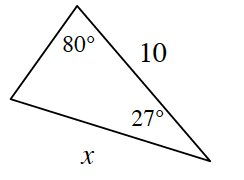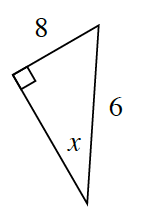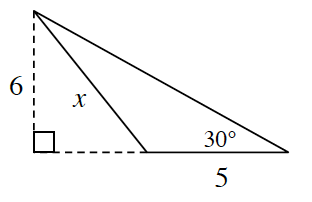Home > CCG > Chapter 9 > Lesson 9.1.4 > Problem9-48

9-48.

For each triangle below, solve for $x$, if possible. If no solution is possible, explain why. Homework Help ✎

1.Which law can you use to solve for $x$?

$180° = 80° + 27° + y$, where $y$ is the unknown angle.

$y = 73°$

Use the Law of Sines to find the length of $x$.

$\frac{\text{sin}80}{x}=\frac{\text{sin}73}{10}$

$x ≈ 10.3$

1.Which side is the longest side of this triangle?
Is that possible?

1.Find the length of the whole base (including the dotted line). How can you use this to find $x$?

$x ≈ 8.07$